https://doi.org/10.5194/amt-16-295-2023
https://doi.org/10.5194/amt-16-295-2023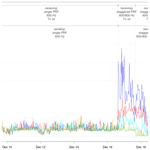Michael Frech, Cornelius Hald, Maximilian Schaper, Bertram Lange, and Benjamin Rohrdantz
Abstract

Share
Dates
1 Introduction

The German weather radar network consists of 17 state-of-the-art dual-pol weather radar systems that are operated at C-band in the frequency range between 5.6 and 5.65 GHz. We operate the DWSR5001SDP/CE radar system of the EEC (Enterprise Electronics Corporation); technical details about this system are given in . The radar network is operated by the German Meteorological Service (Deutscher Wetterdienst, DWD). Weather radars are one of the most important data sources for a wide range of applications. They are the backbone for nowcasting products, which provide reliable warnings to the public in severe weather situations, and they are the only data source to provide quantitative areal information on the precipitation amount and type, which is essential information for hydrological and flood warning applications. Radar data are also assimilated into numerical weather prediction models. The DWD scan strategy has an update frequency of 5 min and consists of two basic scan types. For hydrological applications, a terrain-following scan (referred to as the precipitation scan, PCP) is implemented, where the elevation angle is adjusted as a function of the azimuth angle based on the surrounding orography. This sweep is followed by a volume scan that samples the three-dimensional space at 10 elevations. The volume scan is operated in staggered PRF (pulse repetition frequency), which provides unambiguous Doppler velocities of ±32 m s−1. The parameterizations of those scans are listed in Appendix A. The 5 min sequence ends with a so-called birdbath scan, which is used to calibrate the differential reflectivity .

Before radar data can be used as input for operational products, extensive quality control is needed to identify and mitigate all non-meteorological contributions to the radar signal .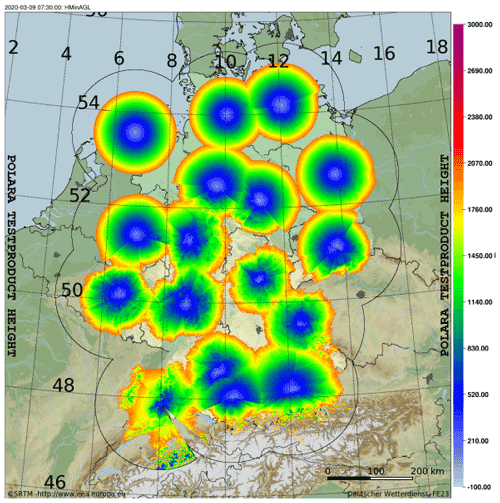Figure 1Radar data coverage up to a height of 2 km above the surface based on the precipitation scan, which is a terrain-following scan. Shown is the coverage of the operational radar network consisting of 17 dual-pol C-band radars. The indicated grid is in degrees latitude north and degrees longitude east.

DWD operates magnetron transmitters with a nominal pulse power of 500 kW. Magnetron transmitters are very common in radar networks all over the world because they are affordable and relatively robust units compared to other available transmitters on the market. The radar design however has to account for some special characteristics of the magnetron tube. The transmit phase for each pulse is random, which means that transmitted pulses are non-coherent. Coherency can be achieved with a reference oscillator. The actual transmit frequency depends on the duty cycle of the magnetron and on temperature. The duty cycle is dependent on the pulse width (PW) and the PRF. The DWD system is operated with a PW of 0.4 and 0.8 µs in a PRF range between 600 and 2410 Hz. With this operating range, the transmit frequency is constant within a range of ± 0.5 MHz. Accounting for typical temperature variations on site (less than 2 K due to air-conditioning), the overall transmit frequency is within ± 1 MHz with respect to the nominal transmit frequency of a magnetron.

Interferences from external sources that operate at C-band are frequently visible in day-to-day operations. Interferences compromise radar data quality, and the problem is expected to increase further . The urgent need to secure and protect atmospheric remote sensing frequency bands is summarized in an overview by . Even though weather radars are the primary user in their band, Wi-Fi devices at C-band are allowed to operate there as well if they use dynamic frequency stepping (DFS). Ideally, DFS should recognize the weather radar in the vicinity and consequently adjust the Wi-Fi transmit frequency to avoid an interference with the weather radar. This works well in theory, but in practice the national frequency authorities (BNetzA, Bundesnetzagentur in Germany) are busy enforcing the proper usage of Wi-Fi devices. A Wi-Fi interference is typically seen as a line-shaped structure in radar products. If the Wi-Fi device is close to the radar, complete sectors of a radar sweep may be affected.

A real-time identification of an interference is implemented in the GAMIC signal processor. It is based on the evaluation of the normalized standard deviation (STD) of the power for a batch of pulses within a ray (ray width is 1), using I/Q (in-phase and quadrature phase) data. Typically, a batch consists of about 60 pulses, which varies depending on the PRF and the rotation speed of the antenna. Internally, I/Q data are sampled to 25 m bins (“raw range bin”). After the signal processing, radar moments are averaged to 250 or 1000 m bins, the so-called processed range bins. A large normalized standard deviation is indicative of the presence of non-coherent power that can be attributed to an external interference. This is because pulsed interferences usually are not correlated with the radar PRF. The standard deviation of the I/Q power magnitude is increased because the interference is expected to be not present in every single pulse. Together with the so-called signal quality index (SQI), which quantifies the coherence of the received signal with the transmitted pulse, an interference contamination can be identified. We check for SQI <0.6 and STD >0.6 to identify an interference. This method is used operationally and proves to be very successful for locating and shutting down interference sources by the frequency authority (see Schaper et al.2022).

In this paper, we assess the performance of the Radar Tango and investigate whether it is possible to introduce additional C-band systems into the radar network. This will be evaluated in terms of the necessary frequency separation to avoid radar–radar interference within the C-band network. Since we need specific design guidelines that work in operational condition with a $\mathrm{24}/\mathrm{7}$ operation of radar systems, an experiment has been designed by using five operational radar systems and the Hohenpeißenberg research radar (MHP) of the German radar network. The allocated transmit frequencies of these operational radar systems cover the C-band frequency range and are therefore well suited for such an experiment. The radars are configured to point directly towards the research radar in order to generate a possible interference. The unique aspect of this setup is that we carried out this dedicated testing over a span of about 4 weeks under quasi-operational conditions. The test was designed in such a way that the operational scanning was not interrupted. This allows for an assessment of the interference situation under a wide range of beam propagation conditions.

As part of the experiment, we also assess the suitability of the Radar Tango as a means of minimizing the radar–radar interference. This is achieved by comparing periods with synchronized and unsynchronized scanning of the Hohenpeißenberg radar with the network radars (Sect. 3.3). The results of these investigations are summarized in the concluding section.

Table 1A summary of operational radar sites used in the interference test. Azimuth () and elevation () describe the angle configuration of the pointing scan towards MHP.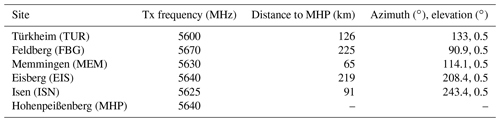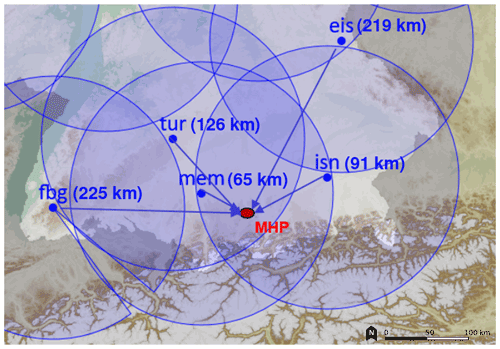Figure 2Location of the radars that are used in this test. Shown are the operational systems with distances to MHP (in blue) and the research radar MHP (in red). Circles have a radius of 150 km. The arrows towards MHP symbolize the interference scan from operational radars.

2 Setup of the interference experiment

Because the operational scanning cannot be interrupted for this experiment, we had to clear approximately 30 s of scanning time at the end of each operational 5 min cycle. To achieve this, it was sufficient to temporarily suspended one of the two birdbath scans (for the two pulse widths).

In order to obtain conclusive results, only one radar at a time is transmitting towards the MHP radar. Using the operational radar software, the set of tests using the five operational systems can be executed in repeating 30 min intervals (Table 2). At the same time, the MHP scheduler is identical to the operational scan, performing the same PCP and volume scan every 5 min. The only difference is the scanning in the 30 s time slot at the end of each 5 min cycle. At MHP, a PCP-like sweep is acquired. All scan configurations can be found in Appendix A.

This test setup was incorporated in the operational scan cycle. In doing so, we were able to capture data under a range of meteorological conditions, especially situations with anomalous propagation (stably stratified atmosphere), but precipitation events were also captured during the test period.

The $\mathrm{24}/\mathrm{7}$ operation of the 30 min scan sequence summarized in Table 2 was initiated on 6 December 2019 and ended on 20 December 2019. During this time period, a number of systematic variations on the transmit (TUR, FBG, MEM, EIS, ISN) and receiving end (MHP) of the test were carried out. On the transmit side there are two periods with a single PRF of 1000 and 600 Hz, respectively. Those periods are followed by a staggered PRF setting of 800 to 600 Hz, which is realized with a time sampling (TS) of 50 pulses (50 pulses with PRF 800 Hz, followed by 50 pulses with a PRF of 600 Hz). On the receiving side, the precipitation scan has a PRF of 600 Hz, or a staggered PRF of 800 to 600 Hz. Additionally, the precipitation scan was operated in receive mode only for some time, where the transmitter of the MHP radar was turned off. The configurations are summarized in Tables 3 and 4.

Table 2The repeating 30 min scan sequence during the interference test. Precipitation scan and volume scan together take about 4 min 30 s to finish and are repeated every 5 min.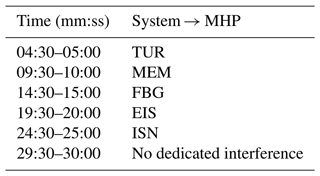Table 3Transmit test configurations from 6–20 December 2019. Operational radars pointing towards radar MHP.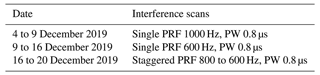It is known that the weather situation has a substantial influence on the propagation of electromagnetic waves in the atmosphere (e.g., Doviak and Zrnic2006). Whether a remote radar can be seen by the MHP radar depends on the static stratification of the atmosphere. This can be assessed by applying a simple yet effective approach for determining the position of a radar beam, the effective earth radius or “$\mathrm{4}/\mathrm{3}$ model” (detailed information and for this and additional comparable models for different atmospheric states can be found in ). The $\mathrm{4}/\mathrm{3}$ model represents a standard atmosphere with a refractivity gradient $\frac{\mathrm{d}N}{\mathrm{d}h}$=42 km−1 .

With this, the height of the beam of a radar over any other point on earth depending on range and elevation angle can be approximated:

$\begin{array}{}\text{(1)}& {H}_{\mathrm{b}}=\sqrt{{r}^{\mathrm{2}}+\left({k}_{\mathrm{e}}\cdot {r}_{\mathrm{e}}{\right)}^{\mathrm{2}}+\mathrm{2}\cdot r\cdot {k}_{\mathrm{e}}\cdot {r}_{\mathrm{e}}\cdot \mathrm{sin}\left(\mathit{\theta }\right)}-{k}_{\mathrm{e}}\cdot {r}_{\mathrm{e}}\phantom{\rule{0.125em}{0ex}}.\end{array}$

Hb is the beam height over ground, r is the range of the beam, ke is $\frac{\mathrm{4}}{\mathrm{3}}$ or the factor for the effective earth radius, re is the earth's radius at the respective latitude, and θ is the elevation angle. The radar EIS will be used as an example for the calculation. The radar EIS is at 49.539 N and 799 $\mathrm{m}\phantom{\rule{0.125em}{0ex}}\mathrm{a}.\mathrm{s}.\mathrm{l}$. The elevation angle θ during the measurements was 0.5, and the distance between the two radars is 219 km. The vertical size of the 3 dB beamwidth at EIS is 0.91, so the (vertical) radius of the beam can be described as

$\begin{array}{}\text{(2)}& {r}_{\mathrm{b},\mathrm{d}}=\left(\mathrm{2}\cdot r\cdot \mathit{\pi }\right)\cdot \frac{{w}_{\mathrm{b}}}{\mathrm{360}}\cdot \frac{\mathrm{1}}{\mathrm{2}}\phantom{\rule{0.125em}{0ex}},\end{array}$

with rb,d being the radius of the beam at distance d and wb being the beam width. This results in a beam radius of 1.739 km at a distance of 219 km. With the $\mathrm{4}/\mathrm{3}$ model, the lower 3 dB edge of the EIS beam over radar MHP (located at 47.793 N, 1000 $\mathrm{m}\phantom{\rule{0.125em}{0ex}}\mathrm{a}.\mathrm{s}.\mathrm{l}.$) is expected to be at 2.1 km. So under normal propagation conditions no interference may be expected. As an estimate, EIS could be visible by MHP with an EIS elevation angle θ= 0 if $\frac{\mathrm{d}N}{\mathrm{d}h}$=150 km−1. This would be achieved with a gradient of about 8 K/100 m. This is not uncommon during clear-sky wintertime high-pressure situations with strong radiative cooling during the night. As is shown later, frequent interferences are detected during the experiment which are attributed to the radar EIS. So periods with strong vertical temperature gradients were present during the experiment.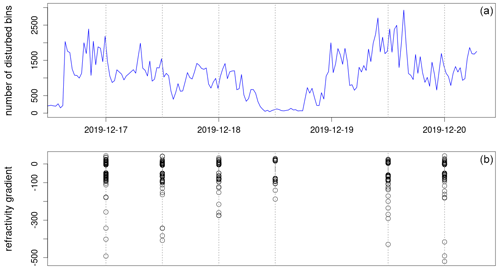Figure 3(a) Number of disturbed bins in the MHP data caused by EIS. (b) Refractivity gradients in the lowest 2.5 km as computed from the Oberschleißheim radiosonde data.

To show this, we have evaluated data from a radiosonde from Oberschleißheim, which is launched twice a day (00:00 and 12:00 UTC). This site is located close to Munich and is almost on the direct line between the radars EIS (distance 156 km) and MHP (distance 65 km) so that the measured vertical temperature gradient is expected to provide a good estimate of the vertical temperature gradient along the beam propagation path. By using the measured temperature, pressure and relative humidity it is possible to approximate the refractivity gradient (details can be found in ). This has been done for the lowest 2.5 km above ground and on the base of dh= 10 m. Figure 3a shows a period in which a highly variable amount of disturbed bins caused by EIS was found (this is a part of the period shown in Fig. 6). The calculated refractivity gradients during the radiosonde ascents is shown in Fig. 3b. During times with few disturbed bins, the refractivity gradients are only slightly negative, while during times with many disturbed bins, the refractivity gradients reach strongly negative values, and the number of strongly negative records is increased.

3 Analysis

All MHP precipitation scans at the end of each 5 min sequence are analyzed for interferences. If the conditions STD >0.6 and SQI <0.6 are satisfied, a range bin is classified as disturbed by an external interference.

In a similar way, interferences are identified from the MHP precipitation scans that happen at the beginning of each 5 min scan cycle as part of the operational scanning schedule. Those results are used to evaluate the Radar Tango in comparison to the MHP precipitation scans that are either synchronized or not with the starting azimuth angle of the other radars.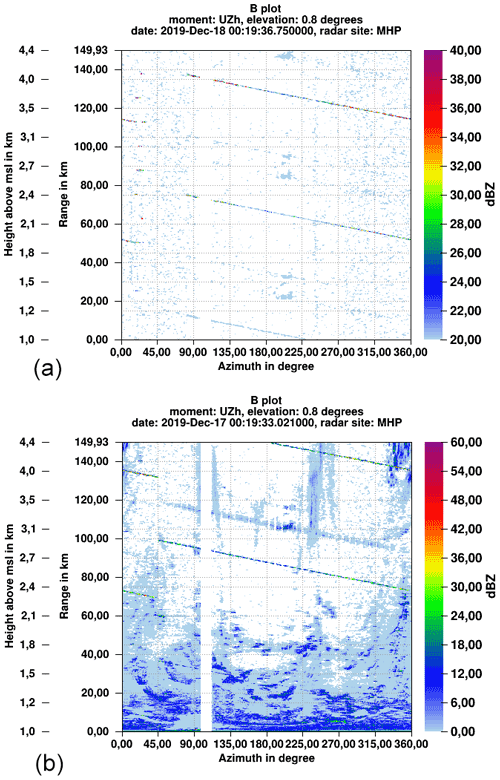Figure 4B plots of uncorrected reflectivity (UZh) illustrating the interference from the Eisberg (EIS) radar (line shape structure). (a) Transmitter turned off, MHP radar only in receive mode. (b) MHP transmitter operating. Corresponding STD B plots are shown in Fig. 5.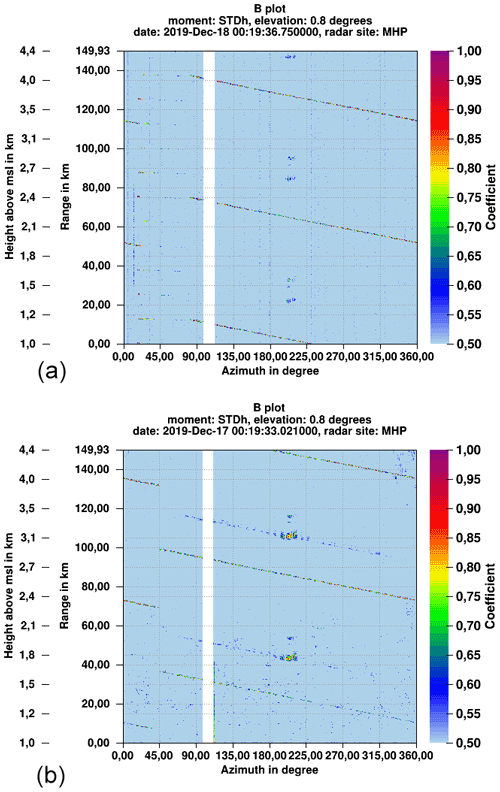Figure 5B plots of the normalized standard deviation of received power STDh (h denoting the horizontal receive channel) illustrating the interference from the Eisberg (EIS) radar (line shape structure). (a) Transmitter turned off, MHP radar only in receive mode. (b) MHP transmitter operating. At MHP, sector blanking is configured between 99 and 111 azimuth because of a church in the safety perimeter.

Before analyzing the results statistically for the different measurement scenarios, we first show some examples. The spiral-shaped signature of radar-induced interference in PPIs can be nicely illustrated in B plots, where the interference then appears as straight lines (Figs. 4 and 5). Figure 4 shows the recorded uncorrected reflectivity (UZh) during two PCP scans within 5 min of each other. The upper image is from a scan where the transmitter at MHP was turned off during the scan, whereas the MHP transmitter was in an operational state in the lower image. In the latter case, clutter power is clearly visible close to the radar. Both images show distinct lines, reaching from the top left to the bottom right. They mark the interference from the EIS radar. The interferences appear at different ranges at the same time, and the lines are parallel to each other. The distance between these lines is determined by the PRFs of the sending and receiving radar. It appears as if the strength of the interference increases with increasing distance from the radar, but this is an artifact of the range correction. The received power is independent of range. Figure 5 shows the STD moment for the two scans described above. Most of the range bins have a STD of ≈0.5 where there is no weather signal and no interference signal. A weather signal typically has values of STD <0.2. In the lines marking the interference from the radar EIS, STD reaches up to 1, showing that the received power within all pulses varies strongly, hinting at a source that is transmitting pulses.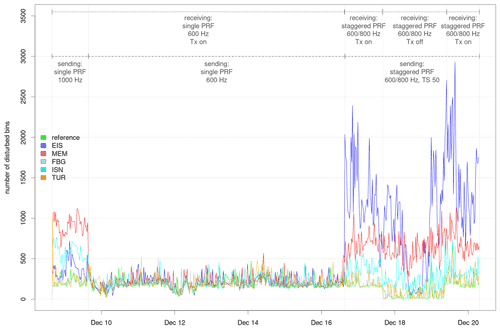Figure 6Time series of the number of pixels with an interference signal caused by the operational radars Eisberg (EIS), Memmingen (MEM), Feldberg (FBG), Isen (ISN) and Türkheim (TUR). Data from the radar Hohenpeißenberg (MHP) are evaluated to identify the interference signal. The dotted line at the top shows the periods of different settings on the receiving MHP radar, the dashed line those of the transmitting radars.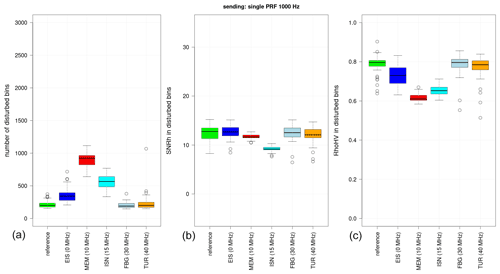Figure 7Boxplot of the number of disturbed bins (a), the SNR in these pixels (b) and the RhoHV in these pixels (c) during the first period as described in Sect. 3.2. The values behind the radar names give their difference in sending frequency relative to the MHP radar.

The second period starts at the end of the first and ends on 16 December 2019 at 15:00 UTC. During this time, both the receiving radar and the transmitting radars are operated at a single PRF of 600 Hz. This PRF combination obviously has the smallest number of interferences. Unless the transmitted pulses are exactly synchronized in time, there is only a small chance to capture the transmitted pulse from one of the external radars of this experiment. However, the pulse trigger timing cannot be synchronized because the trigger timing of a particular scan depends on the timing of the previous scans, which involves for example the time it takes to position the antenna. This will be variable from system to system. Another aspect to keep in mind is that the receiving PCP scan only samples data up to a range of 150 km, whereas the unambiguous range is 250 km. So any pulse which would appear in a range beyond 150 km cannot be captured on the receive side. However with different PRF settings, there always will be pulses that are captured by the MHP system within the scan time of 30 s.

Variations during the second period are caused by small changes in the elevation of the transmitting radars and by changes in weather, causing different conditions for propagation. The evaluation of SNR and RhoHV for this period (not shown) confirms these findings with no discernible differences among the radars. Most of the identified interferences presumably originate from Wi-Fi routers and not from the other radars.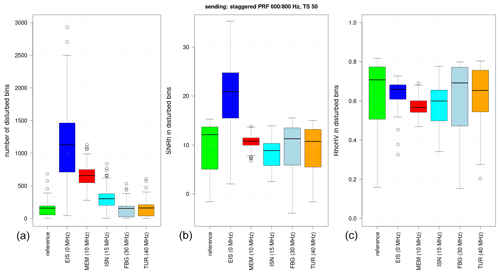Figure 8Boxplot of the number of disturbed bins (a), the SNR in these pixels (b) and the RhoHV in these pixels (c) during the third period as described in Sect. 3.2. The values behind the radar names give their difference in sending frequency relative to the MHP radar.

The corresponding boxplot for the whole third period is shown in Fig. 8, which again illustrates that the number of disturbed pixels is dependent on transmit frequency. The largest SNR values are measured when the transmit frequencies exactly match (EIS/MHP). Median SNR is then on the order of 20 dB. SNR reaches similar levels of about 14 dB for all other radars and the reference data set. The already-mentioned frequency dependence is also revealed by the increased number of interference pixels within the 15 MHz band from the MHP radar. RhoHV values are between 0.6 and 0.8, which is indicative of a non-meteorological signal. Also, the variability in RhoHV values is much higher than in the first period. This is due to the fact that RhoHV is a function of SNR. At low SNR with SNR < 10 dB, RhoHV never reaches values above 0.85, even in pure rain. For further details we refer to Ryzhkov and Zrnic (2019, Sect. 6.7). The third period has many bins with low SNR values, causing low RhoHV values in return.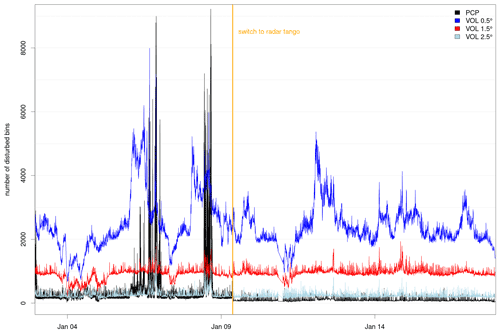Figure 9Time series of interference bins in the MHP PCP scan and the lowest three elevations of the VOL scan. The orange line marks the beginning to the Radar Tango.

One reason for the relatively large interference numbers in the lower elevations of the VOL scan is attributed to the surrounding orography: when the radar is pointing towards the Alpine ridge in the south at low elevation, the chance of detecting an external microwave source is increased. The elevation adjustments of the PCP (see Table A2) to avoid blocking from the orography effectively avoid sources of interference (Fig. 9). An increase of already 1 in elevation substantially decreases the number of range bins with an interference signal.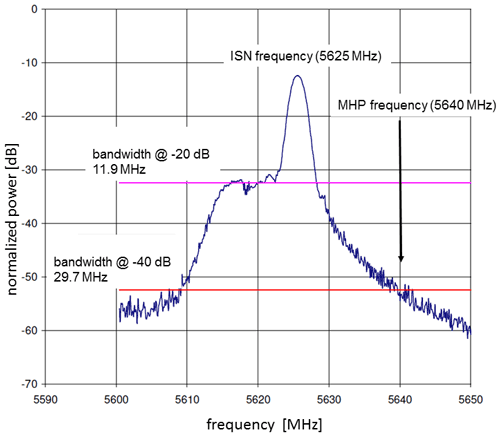Figure 10Measured frequency spectrum of the ISN magnetron for a pulse length of 0.4 µs. Measurements were taken between the magnetron and the wave guide filters. The resulting bandwidths at 20 and 40 dB are shown. The ISN transmitter is operated at 5625 MHz. For illustrative purposes, the MHP transmitter frequency (5640 MHz) is shown as well.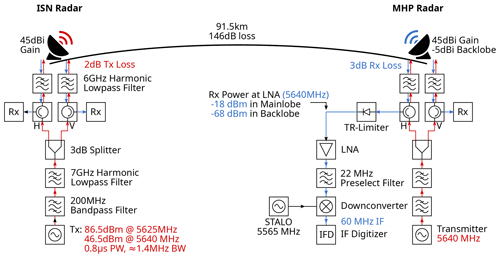Figure 11Radar setup used for the analysis. The transmit signal from the ISN radar at 5640 MHz interferes with the Rx at the MHP radar at 5625 MHz. Important radio frequency components are included for reference.

## 3.4 Discussion

Based on the previous analysis, radars separated by more than 15 MHz in transmit frequency from the MHP radar cause no interference signal. In addition, the Radar Tango proves to be an effective method to avoid radar–radar interference. The plausibility of this is now discussed by considering the effect of the transmitter and receiver components on the characteristic spectrum of a magnetron pulse with a given bandwidth. The following is illustrated with Fig. 11.

$\begin{array}{}\text{(3)}& {P}_{\mathrm{Rx}}={P}_{\mathrm{Tx}}\left({f}_{\mathrm{Rx}}\right)-\text{FSPL}+{G}_{\mathrm{Tx}}+{G}_{\mathrm{Rx}}-{A}_{\mathrm{G}}-{L}_{\mathrm{Tx}}-{L}_{\mathrm{Rx}}\phantom{\rule{0.125em}{0ex}},\end{array}$

with FSPL being the free-space path loss; AG the gas attenuation; LTx and LRx the transmit and receive losses, respectively; and GRx and GTx the antenna gain of the receiving and transmitting antenna. The term PTx at fRx refers to the transmitted power of the magnetron in the nominal operating frequency band of the receiving radar. The FSPL (dB) can be written as

$\begin{array}{}\text{(4)}& \text{FSPL}=\mathrm{10}{\mathrm{log}}_{\mathrm{10}}{\left(\frac{\mathrm{4}\mathit{\pi }d}{\mathit{\lambda }}\right)}^{\mathrm{2}}\phantom{\rule{0.125em}{0ex}},\end{array}$

with the radar wavelength λ and the distance d between the two radars.

We consider the radar ISN, which operates at 5625 MHz and is 91 km away from the radar Hohenpeißenberg. We now want to compute the expected power at the MHP low-noise amplifier (LNA) using the measured emitted power of the ISN magnetron at the MHP radar frequency 5640 MHz. A measured spectrum of a 0.4 µs pulse is shown in Fig. 10. For a pulse length of 0.8 µs the 3 dB bandwidth is smaller, but the overall shape is similar. The measured bandwidth of the ISN pulse at the 40 dB level (40 dB below the peak) is about 30 MHz. Even though the pulse is not strictly symmetric, the power spectral density at 5640 MHz is about 40 dB below the peak at 5625 MHz. The total transmit power is about 86.5 dBm and is reduced by approximately 2 dB of transmit losses and another 3 dB for the power splitter. The major part of the power is concentrated in the desired 1.4 MHz band around 5625 MHz. In the same bandwidth at 5640 MHz, a total power of roughly 44 dBm ($\mathrm{86.5}-\mathrm{2}-\mathrm{40}$) can be estimated. Antenna gain on the transmit and receive path is 45 dB. With a receive loss of 3 dB and the given distance of 91.5 km, which results in a FSPL of 146 dB, we can approximate about 18 dBm of power from the ISN radar in the matched filter bandwidth of the MHP radar in the LNA reference plane. This would result in a relatively strong signal which is well within the linear sensitivity range of the receiver (110 to 7 dBm). The results represent a worst-case scenario, since the two radars do not have a free line of sight, and the total signal loss on the transmission path will be significantly higher in reality. Gas attenuation has a negligible effect amounting to 0.7 dB. Note that it is not necessary to consider the waveguide filters because their relatively large bandwidth does not significantly alter the analyzed part of the pulse spectrum.

For the Radar Tango, a similar estimate can be made by assuming a gain of 5 dB (back lobe of the antenna) on the receiving system and a gain of 45 dB for the transmitting system. The other specifications remain the same. It is important to realize that this is the case for just one azimuth angle when a radar pair is perfectly aligned with free line of sight. For the precipitation scan (12${}^{\circ }\phantom{\rule{0.125em}{0ex}}{\mathrm{s}}^{-\mathrm{1}}$ antenna speed) this occurs for about 0.08 s. For this special configuration, a power of 66 dBm could be expected at the MHP LNA. According to the antenna diagram (see Frech et al.2013), the expected power drops below 100 dBm within about 3. This is because antenna side lobes are below 40 to 50 dB beyond 3. Outside the perfect radar pair alignment we then can assume a gain of 5 dB for both antennas. For this simplified scenario we could expect 118 dBm at the LNA of the MHP radar, which is well below the sensitivity level.

This simple back-of-the-envelope calculation illustrates that a combination of radar siting, orography and frequency spacing helps to minimize radar interference. The most effective mitigation of interference however can be achieved by the Radar Tango. Except when there is a perfect alignment of the radars, the expected power at the LNA is below the radar sensitivity. Interferences in the situation of a perfect alignment in azimuth are not seen in day-to-day operations. This can be attributed to the fact that all radars scan at an elevation ≥0.5 so that only the power from side lobes may play a role. Then the corresponding power levels are small (see above) so that there is no interference even during the brief instances of perfect alignment. This is supported by the measurements in this study and from the day-to-day experience after the introduction of the Radar Tango in the DWD radar network.

4 Summary

• As an obvious result, interference is strongest when both the transmitting and the receiving radar operate at the same transmit frequency.

• The interference signal from an external source is only seen up to a transmit frequency separation of about 15 MHz. In our case, this result is found for the radar ISN which operates at 5625 MHz. This result may vary for a different magnetron transmitter/filter setup.

• For identical frequencies, SNR is on average near 20 dB. For all other frequencies, SNR is about 14 dB, while there is a strong frequency dependence with respect to the number of range bins with an interference signal. Time series of interference pixel numbers show a lot of variability in the number of range bins with interference, which relates to variability in propagation effects and, to a minor extent, the random sampling of the interference by the MHP radar.

• When allocating a transmit frequency, radars that are over 200 km away have to be included in the considerations. This is illustrated by the clear interference signal in the MHP radar data caused by the radar EIS at 219 km distance.

• The Radar Tango is the most effective means to minimize the radar–radar interference (up to 50 dB higher suppression). Time periods with an increased number of disturbed pixels are found when the radars are not synchronized every 5 min to start with the same azimuth angle.

• The relatively broad frequency spectrum of a typical magnetron pulse may cause interference in a large frequency range within the usable C-band. This issue can be efficiently mitigated by the Radar Tango.

To summarize, these results provide a guidance to determine a proper frequency assignment in a C-band weather radar network such that the possibility of a radar–radar-induced interference is minimized. When selecting the appropriate frequencies, even radars at a distance around 200 km have to be considered since they have a chance to become visible to a radar (in our case the EIS-MHP radar pair) in stably stratified situations with favorable refractive gradients. An increased number of interferences is found up to a frequency separation of 15 MHz. Beyond this limit, the number of range bins with an interference signal is comparable to data where no dedicated external interference signal is present.

Appendix A: Scan parameterizations

The parameterizations of the operational DWD precipitation (PCP) and volume (VOL) scans used during this investigation are shown in Tables A1, A2 and A3, respectively. Note that the range bin resolution in the volume scan was increased in spring 2021 from 1 km to 250 m.

Table A1The scan parameterization of the operational precipitation (PCP) scan.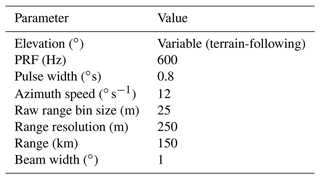Table A2Elevation angles as a function of azimuth angle of the operational PCP scan at MHP.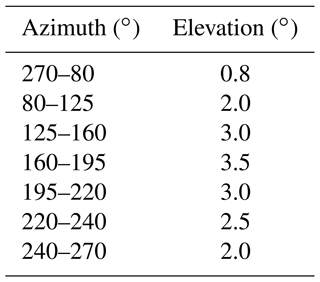Table A3Scanning parameters of the operational volume (VOL) scan. Two values for PRF implies staggering.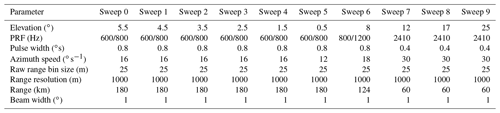Data availability

Data sets used for this study are available upon request.

Author contributions

MF designed the experiment and carried out the measurements and parts of the analysis. The main portions of the article were written by MF. CH analyzed the data and wrote parts of the article. MS implemented the interference detection, ML contributed to the technical description of the radar system, and BR and BL contributed to the analysis and interpretation of the results.

Competing interests

The contact author has declared that none of the authors has any competing interests.

Disclaimer

Publisher's note: Copernicus Publications remains neutral with regard to jurisdictional claims in published maps and institutional affiliations.

Acknowledgements

The fruitful discussions with the DWD radar development team are gratefully acknowledged. We want to acknowledge in particular the significant contribution of Theo Mammen and Kay Desler. Their exceptional work formed the basis for this study. We also acknowledge the constructive and helpful comments of the reviewers, which helped to improve the paper.

Review statement

This paper was edited by Gianfranco Vulpiani and reviewed by two anonymous referees.

References

Doviak, R. J. and Zrnic, D. S.: Doppler Radar and Weather Observations, Dover Publications, Inc, 2006. a

Frech, M. and Hubbert, J.: Monitoring the differential reflectivity and receiver calibration of the German polarimetric weather radar network, Atmos. Meas. Tech., 13, 1051–1069, https://doi.org/10.5194/amt-13-1051-2020, 2020. a

Frech, M., Lange, B., Mammen, T., Seltmann, J., Morehead, C., and Rowan, J.: Influence of a Radome on Antenna Performance, J. Atmos. Ocean. Tech., 30, 313–324, 2013.  a

Frech, M., Hagen, M., and Mammen, T.: Monitoring the Absolute Calibration of a Polarimetric Weather Radar, J. Atmos. Ocean. Tech., 34, 599–615, https://doi.org/10.1175/JTECH-D-16-0076.1, 2017. a

Holleman, I. and Huuskonen, A.: Analytical formulas for refraction of radiowaves from exoatmospheric sources, Radio Sci., 48, 226–231, https://doi.org/10.1002/rds.20030, 2013. a

ITU-R: RECOMMENDATION ITU-R RS.1280: Selection of active spaceborne sensor emission characteristics to mitigate the potential for interference to terrestrial radars operating in frequency bands 1–10 GHz, Tech. rep., ITU, https://extranet.itu.int/ (last access: 11 January 2023), 1997. a

Martin, G. and Vaclav, K.: Atmospheric Refraction and Propagation in Lower Troposphere, in: Electromagnetic Waves, editied by: Zhurbenko, V., InTech, 140–156, ISBN: 978-953-307-304-0, https://www.intechopen.com/chapters/16079 (last access: 11 January 2023), 2011. a, b

Palmer, R., Whelan, D., Bodine, D., Kirstetter, P., Kumjian, M., Metcalf, J., Yeary, M., Yu, T.-Y., Rao, R., Cho, J., Draper, D., Durden, S., English, S., Kollias, P., Kosiba, K., Wada, M., Wurman, J., Blackwell, W., Bluestein, H., Collis, S., Gerth, J., Tuttle, A., Wang, X., and Zrnić, D.: The Need for Spectrum and the Impact on Weather Observations, B. Am. Meteorol. Soc., 102, E1402–E1407, https://doi.org/10.1175/BAMS-D-21-0009.1, 2021. a

Ryzhkov, A. V. and Zrnic, D. S.: Radar polarimetry for weather observations, Vol. 486, Springer, Cham, Switzerland, https://doi.org/10.1007/978-3-030-05093-1, 2019. a

Saltikoff, E., Cho, J. Y. N., Tristant, P., Huuskonen, A., Allmon, L., Cook, R., Becker, E., and Joe, P.: The Threat to Weather Radars by Wireless Technology, B. Am. Meteorol. Soc., 97, 1159–1167, https://doi.org/10.1175/BAMS-D-15-00048.1, 2016. a

Schaper, M., Frech, M., Michaelis, D., Hald, C., and Rohrdantz, B.: Radio frequency interference detection and mitigation in the DWD C-band weather radar network, Atmos. Meas. Tech., 15, 6625–6642, https://doi.org/10.5194/amt-15-6625-2022, 2022. a, b

Werner, M. and Steinert, J.: New quality assurance algorithms the DWD polarimetric C-band weather radar network, in: 7th Europ. Conf. On Radar in Meteor. and Hydrol., Toulouse, France, 24–29 June 2012, NET403, http://www.meteo.fr/cic/meetings/2012/ERAD/extended_abs/NET_403_ext_abs.pdf (last access: 11 January 2023), 2012. a